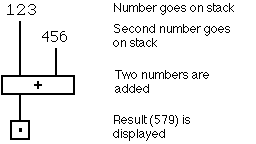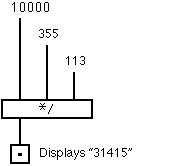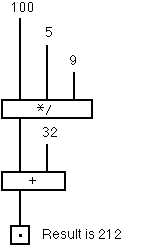# Using Forth's Command Line Interpreter

The most common Forth interface is a command line interpreter. Forth recognises a large number of instructions (called "words"). Here we will look at a small selection of them.

The word "`+`" adds two integers. It is used like this;
(Words in Forth are delimited by spaces.)```   123 456 + . [cr]  579 ok
```
Where [cr] means press "enter", and the emphasised text is the computers response.

The period "." is another Forth word (called "dot"), that prints a number.Forth has an exceptionally rich set of integer operators, as it is often used on systems that do not support floating point very well. An unusual one is "`*/`", which is a scaling operator. It's stack comment is `( n1 n2 n3--n4)`, where the result (n4) is n1 multiplied by n2 and divided by n3.

To test this we might try

```   10000 355 113 */ . [cr] 31415 ok
```
and thus discover that 355/113 is a good approximation to pi.More than one word can be issued at a time, so as an example, to convert from degrees Celsius to degrees Fahrenheit, we take nine fifths, and add thirty two. This can be tested thus.
```   100 9 5 */ 32 + . [cr] 212 ok
```
The converse operation, converting from degrees Fahrenheight to degrees Celsius is left as an exercise for the reader.

(But as a hint, the word to subtract one number from another is "`-`". It's stack comment is ( n1 n2--n3), where n3 is n1-n2)

Now that you know almost all there is to know about the Forth command line interpreter, and have learned a few maths operators as well, you can return to the contents page, or go on to the next section.